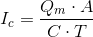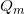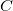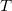# Chemical Injection Rate (Mass)

Calculates the chemical injection rate by mass, such as when the application rate is specified in pounds per acre (common with fertilizers). Learn more about the units used on this page. For more information go here for a fact sheet on how to calculate chemigation injection rates for weight or mass-based targets (example: lbs/acre).

Desired Application in mass/area:
Area to be Applied to:
Injected Solution Concentration:
Injection Time:
Calculated Chemical Injection Rate:

## The Equation

When calculating the chemical injection rate by mass use this formula.When:= The chemical injection rate in gallons per hour (gph)= The quantity of chemical to be applied by mass in pounds per acre (lbs/acre)= The concentration of the solution injected, sometimes listed on the label as active ingredient. Measured in pounds per gallon (lbs/gal).= The amount of time the chemical solution is injected in hours (hrs).

WSU Prosser - IAREC, 24106 N Bunn Rd, Prosser WA 99350-8694, 509-786-2226,Contact Us